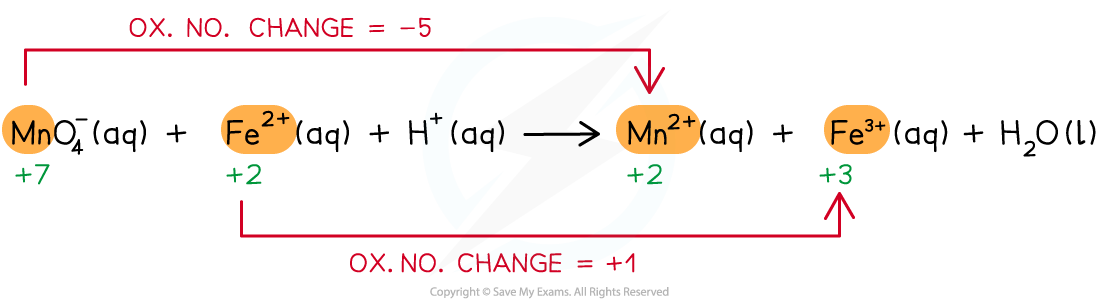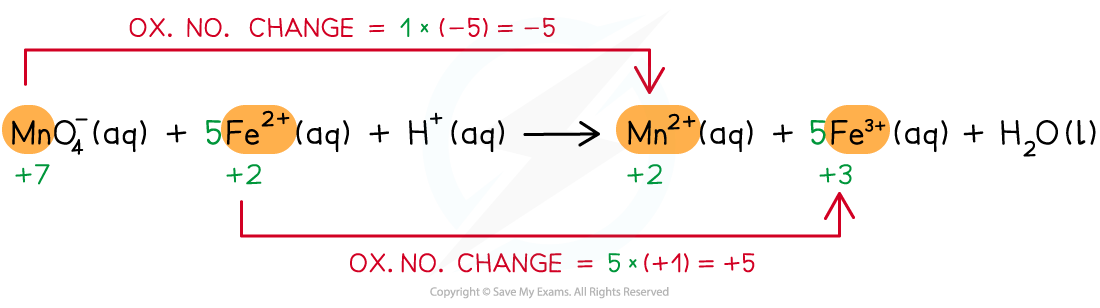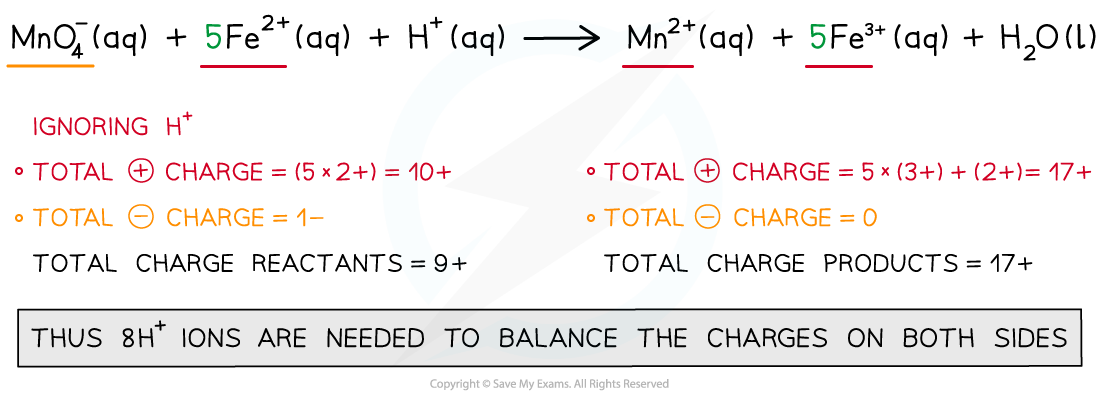# IB DP Chemistry: SL复习笔记9.1.5 Half Equations

### Half Equations

• Oxidation numbers can be used to balance chemical equations
• Go through these steps to balance a redox equation:
1. Write the unbalanced equation and identify the atoms which change in ox. no.
2. Deduce the ox.no. changes
3. Balance the ox.no. changes
4. Balance the charges
5. Balance the atoms

#### Worked Example

Manganate(VII) ions (MnO4-) react with Fe2+ ions in the presence of acid (H+) to form Mn2+ ions, Fe3+ ions and waterWrite the overall redox equation for the reaction

Step 1: Write the unbalanced equation and identify the atoms which change in ox. no.Step 2: Deduce the ox.no. changesStep 3: Balance the ox.no. changesStep 4: Balance the chargesStep 5: Balance the atoms